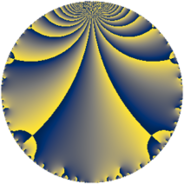# Properties

 Label 169.4.aLevel $169$ Weight $4$ Character orbit 169.a Rep. character $\chi_{169}(1,\cdot)$ Character field $\Q$ Dimension $33$ Newform subspaces $12$ Sturm bound $60$ Trace bound $3$

# Related objects

## Defining parameters

 Level: $$N$$ $$=$$ $$169 = 13^{2}$$ Weight: $$k$$ $$=$$ $$4$$ Character orbit: $$[\chi]$$ $$=$$ 169.a (trivial) Character field: $$\Q$$ Newform subspaces: $$12$$ Sturm bound: $$60$$ Trace bound: $$3$$ Distinguishing $$T_p$$: $$2$$

## Dimensions

The following table gives the dimensions of various subspaces of $$M_{4}(\Gamma_0(169))$$.

Total New Old
Modular forms 53 44 9
Cusp forms 39 33 6
Eisenstein series 14 11 3

The following table gives the dimensions of the cuspidal new subspaces with specified eigenvalues for the Atkin-Lehner operators and the Fricke involution.

$$13$$Dim.
$$+$$$$18$$
$$-$$$$15$$

## Trace form

 $$33 q + 4 q^{2} + 2 q^{3} + 110 q^{4} + 10 q^{5} - 12 q^{6} + 22 q^{7} + 48 q^{8} + 159 q^{9} + O(q^{10})$$ $$33 q + 4 q^{2} + 2 q^{3} + 110 q^{4} + 10 q^{5} - 12 q^{6} + 22 q^{7} + 48 q^{8} + 159 q^{9} - 78 q^{10} - 54 q^{11} + 150 q^{12} - 186 q^{14} - 16 q^{15} + 274 q^{16} - 98 q^{17} + 220 q^{18} + 210 q^{19} + 100 q^{20} + 212 q^{21} - 136 q^{22} - 144 q^{23} - 588 q^{24} + 581 q^{25} - 520 q^{27} + 96 q^{28} + 188 q^{29} + 226 q^{30} - 110 q^{31} + 208 q^{32} - 76 q^{33} + 520 q^{34} - 164 q^{35} - 258 q^{36} - 78 q^{37} - 482 q^{38} - 202 q^{40} - 106 q^{41} + 220 q^{42} - 226 q^{43} + 620 q^{44} + 334 q^{45} - 476 q^{46} - 330 q^{47} - 72 q^{48} - 211 q^{49} - 236 q^{50} + 184 q^{51} + 380 q^{53} + 84 q^{54} - 212 q^{55} + 344 q^{56} - 1488 q^{57} - 1204 q^{58} + 662 q^{59} - 1008 q^{60} + 916 q^{61} + 1706 q^{62} + 1846 q^{63} - 908 q^{64} + 2406 q^{66} - 546 q^{67} - 2148 q^{68} - 1844 q^{69} + 580 q^{70} + 122 q^{71} - 360 q^{72} - 554 q^{73} - 1256 q^{74} + 670 q^{75} + 2120 q^{76} - 1148 q^{77} - 148 q^{79} + 692 q^{80} - 839 q^{81} + 2070 q^{82} - 1650 q^{83} - 2956 q^{84} + 712 q^{85} - 2364 q^{86} + 1152 q^{87} - 120 q^{88} + 1910 q^{89} - 2740 q^{90} + 3208 q^{92} + 720 q^{93} - 478 q^{94} - 1092 q^{95} + 1268 q^{96} + 858 q^{97} - 220 q^{98} + 702 q^{99} + O(q^{100})$$

## Decomposition of $$S_{4}^{\mathrm{new}}(\Gamma_0(169))$$ into newform subspaces

Label Dim $A$ Field CM Traces A-L signs $q$-expansion
$a_{2}$ $a_{3}$ $a_{5}$ $a_{7}$ 13
169.4.a.a $1$ $9.971$ $$\Q$$ None $$-4$$ $$2$$ $$-17$$ $$-20$$ $+$ $$q-4q^{2}+2q^{3}+8q^{4}-17q^{5}-8q^{6}+\cdots$$
169.4.a.b $1$ $9.971$ $$\Q$$ None $$-3$$ $$-1$$ $$9$$ $$-15$$ $-$ $$q-3q^{2}-q^{3}+q^{4}+9q^{5}+3q^{6}-15q^{7}+\cdots$$
169.4.a.c $1$ $9.971$ $$\Q$$ None $$3$$ $$-1$$ $$-9$$ $$15$$ $-$ $$q+3q^{2}-q^{3}+q^{4}-9q^{5}-3q^{6}+15q^{7}+\cdots$$
169.4.a.d $1$ $9.971$ $$\Q$$ None $$4$$ $$2$$ $$17$$ $$20$$ $+$ $$q+4q^{2}+2q^{3}+8q^{4}+17q^{5}+8q^{6}+\cdots$$
169.4.a.e $1$ $9.971$ $$\Q$$ None $$5$$ $$-7$$ $$7$$ $$13$$ $+$ $$q+5q^{2}-7q^{3}+17q^{4}+7q^{5}-35q^{6}+\cdots$$
169.4.a.f $2$ $9.971$ $$\Q(\sqrt{17})$$ None $$-5$$ $$5$$ $$-15$$ $$15$$ $+$ $$q+(-2-\beta )q^{2}+(1+3\beta )q^{3}+5\beta q^{4}+\cdots$$
169.4.a.g $2$ $9.971$ $$\Q(\sqrt{17})$$ None $$-1$$ $$5$$ $$3$$ $$9$$ $+$ $$q-\beta q^{2}+(4-3\beta )q^{3}+(-4+\beta )q^{4}+\cdots$$
169.4.a.h $2$ $9.971$ $$\Q(\sqrt{3})$$ None $$0$$ $$-14$$ $$0$$ $$0$$ $-$ $$q+2\beta q^{2}-7q^{3}+4q^{4}+8\beta q^{5}-14\beta q^{6}+\cdots$$
169.4.a.i $2$ $9.971$ $$\Q(\sqrt{3})$$ None $$0$$ $$4$$ $$0$$ $$0$$ $-$ $$q+\beta q^{2}+2q^{3}-5q^{4}+\beta q^{5}+2\beta q^{6}+\cdots$$
169.4.a.j $2$ $9.971$ $$\Q(\sqrt{17})$$ None $$5$$ $$5$$ $$15$$ $$-15$$ $+$ $$q+(3-\beta )q^{2}+(4-3\beta )q^{3}+(5-5\beta )q^{4}+\cdots$$
169.4.a.k $9$ $9.971$ $$\mathbb{Q}[x]/(x^{9} - \cdots)$$ None $$-5$$ $$1$$ $$-30$$ $$-38$$ $-$ $$q+(-1+\beta _{1})q^{2}+\beta _{2}q^{3}+(5+\beta _{5}+\beta _{6}+\cdots)q^{4}+\cdots$$
169.4.a.l $9$ $9.971$ $$\mathbb{Q}[x]/(x^{9} - \cdots)$$ None $$5$$ $$1$$ $$30$$ $$38$$ $+$ $$q+(1-\beta _{1})q^{2}+\beta _{2}q^{3}+(5+\beta _{5}+\beta _{6}+\cdots)q^{4}+\cdots$$

## Decomposition of $$S_{4}^{\mathrm{old}}(\Gamma_0(169))$$ into lower level spaces

$$S_{4}^{\mathrm{old}}(\Gamma_0(169)) \cong$$ $$S_{4}^{\mathrm{new}}(\Gamma_0(13))$$$$^{\oplus 2}$$